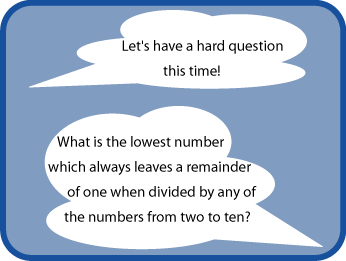#### You may also like### Exploring Wild & Wonderful Number Patterns

EWWNP means Exploring Wild and Wonderful Number Patterns Created by Yourself! Investigate what happens if we create number patterns using some simple rules.### Sending Cards

This challenge asks you to investigate the total number of cards that would be sent if four children send one to all three others. How many would be sent if there were five children? Six?### Dice and Spinner Numbers

If you had any number of ordinary dice, what are the possible ways of making their totals 6? What would the product of the dice be each time?

# Divide it Out

## Divide it Out### Why do this problem?

This problem requires logical thinking about factors and much multiplication! Learners must first find out what is the lowest number which divides exactly by all the numbers from $2$ to $10$ and then decide how this helps to answer the question asked. A calculator could be useful for checking.

### Key questions

How are you going to start on this problem?
Why not start by listing all the numbers from $2$ to $10$?
Which numbers do you not need because they are factors of other numbers you are using?

### Possible extension

Learners could find the lowest number which always leaves a remainder of $1$ when divided by any of the numbers from $2$ to $12$, or $2$ to $20$, etc.

### Possible support

Suggest starting by finding the lowest number which divides exactly by all the numbers from $2$ to $6$. A calculator might be very helpful.